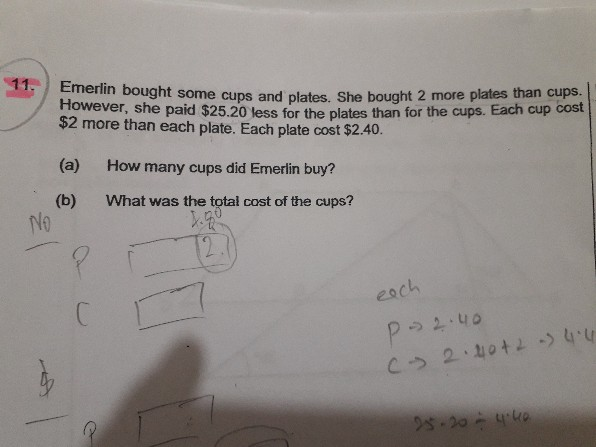QuestionCan any one help to solve this problem clearly in model and also in ratio

Source: ?

Plates

Qty =U + 2, price/piece= \$2.40,

total cost = 2.40 x (U+2) = 2.40U + 4.80

Cups

Qty = U, Price/piece =\$4.40, total cost = 4.40U

4.40U – (2.40U + 4.80) = 25.20

4.40U – 2.40U – 4.80  = 25.20

2U  – 4.80 = 25.20

2U= 30, 1U = 15

a) cups bought = 15

b) Price of cups = 15 x 4.40 = 66\$

0 Replies 0 Likes